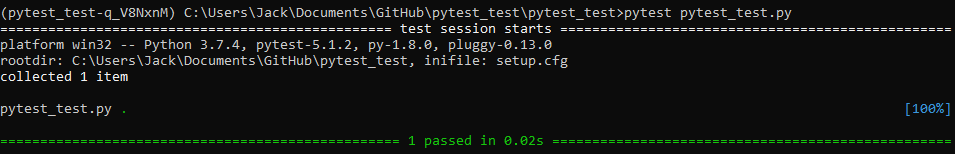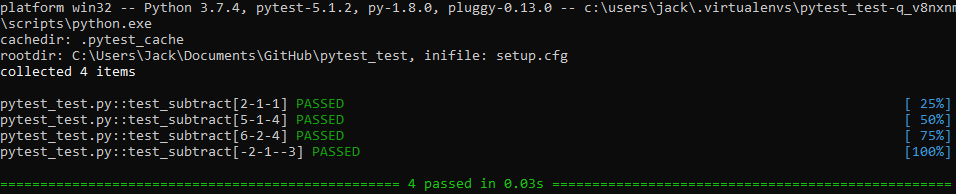# Introduction to Pytest & Pipenv

Posted on Fri 20 September 2019 in Python • 2 min read

Unit tests in general are good practice within software development, they are typically automated tests written to ensure that a function or section of a program (a.k.a the 'unit') meets its design and behaves as intended.

This post won't go into testing structures for complex applications, but rather just a simple introduction on how to write, run and check the output of a test in Python with pytest.

As this post is on testing, I also thought it might be quite apt for trialing out a difference package for dependency management. In the past I've used anaconda, virtualenv and just pip, but this time I wanted to try out pipenv.

Similar to my post Python Project Workflow where I used virtualenv, you must install pipenv in your base Python directory, and typically add the Scripts folder to your path for ease later on. Now all we need to do is navigate to the folder and run:

 `1` ``````pipenv shell ``````

This will create a virtual environment somewhere on your computer (unless specified) and create a pipfile in the current folder. The pipfile is a file that essentially describes all the packages used within the project, their version number & so on. This is extremely useful when you pick it back up later on and find where you were at or if you wish to share this with others, they can generate their own virtual environment simply from the pipfile with:

 `1` ``````pipenv install --dev ``````

Enough about pipenv, let's get onto trying out pytest.

For this post I will place both my function and it's tests in the same file, however, from my understanding it's best practice to separate them, specifically keeping all tests within an aptly named 'tests' directory for your project/package.

First off let's define the function we intend to test later:

 ```1 2``` ``````def subtract(number_1, number_2): return number_1 - number_2 ``````

Now we want to test if our function returns 1 if we give it number_1 = 2 and number_2 = 1:

 ```1 2 3 4``` ``````import pytest def test_subtract(): assert subtract(2,1) == 1 ``````

To run this test, open the pipenv shell like above in the directory of the file where you've written your tests and run:

 `1` ``````pytest file_name.py ``````

This will output the following:Each green dot represents a single test, and we can see that our 1 test passes in 0.02 seconds.

To get more information from pytest, use the same command with -v (verbose) option:

 `1` ``````pytest file_name.py -v ``````

Now we might want to check that it works for multiple cases, to do this we can use the parametrize functionality of pytest like so:

 ``` 1 2 3 4 5 6 7 8 9 10 11 12 13``` ``````import pytest def subtract(number_1, number_2): return number_1 - number_2 @pytest.mark.parametrize('number_1, number_2, expected', [ (2,1,1), (5,1,4), (6,2,4), (-2,1,-3), ]) def test_subtract(number_1,number_2,expected): assert expected == subtract(number_1,number_2) ``````

Once run with the verbose command, we get the output:Hopefully this post is a gentle introduction to what unit testing can be in Python.